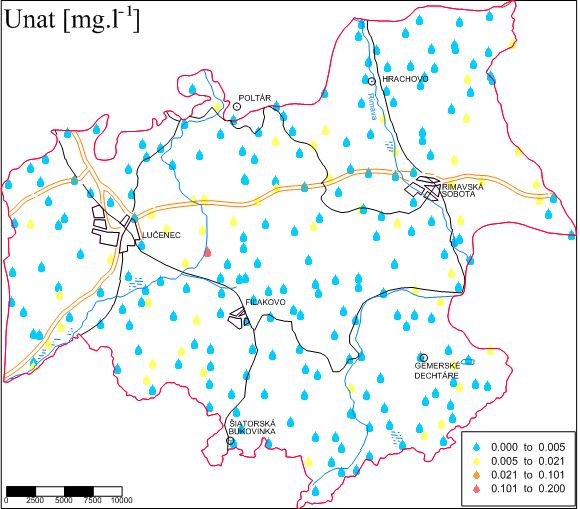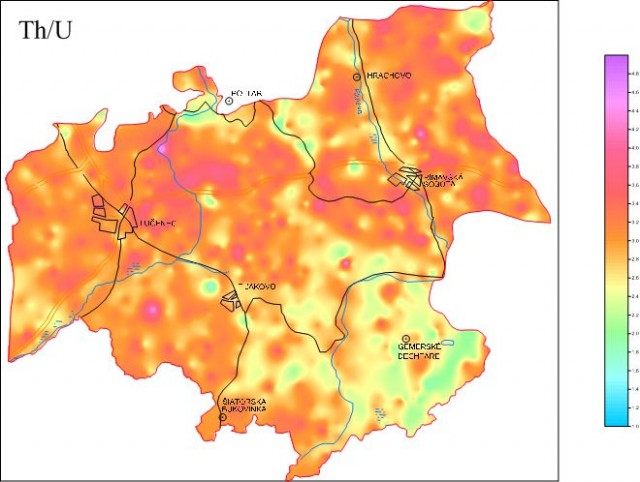Radiometric methods are based on measurements of the emitted radiation by radioactive elements contained in rock complexes.

Geological mapping
Search for uranium and precious metals
Tectonics mapping
Geofactors of the environment

Theory

Radioactivity is one of the aspects of the environment throughout the duration of the planet. The entire biosphere during its existence has been permanently located in the radiation field. Radioactive burden is caused by extraterrestrial and terrestrial sources (these can be further divided into natural and artificial). According to the mode of action for humans radioactive field can be divided into external and internal.
Radioactivity is a phenomenon in which the nuclei of atoms of an element spontaneously convert to the core of the other component, the high-energy radiation is emitted. Nuclei showing this property are called radionuclides.
Radioactivity in rocks is mainly caused by (from more than 230 radionuclides) 40K, 238U, 232Th. Thorium and uranium produce natural radioactive decay series whose items are unstable and act as sources of radiation. An important item of these decay series is a gaseous element radon.

Potassium 40K is a widespread natural (primary) radionuclide with a very long half-life 1,26.109 years.

Uranium 238U (T1 / 2 = 4,51.109 years) is due to its longer half-life one of the most widespread type of uranium in nature. It can also be used as fissile material, but not directly, but through plutonium, which arises in a nuclear reactor from the 238U absorption of neutrons.

Thorium 232Th (T1 / 2 = 1,39.1010 years) is one of the most common natural radionuclides contained in the rocks of the crust (with 40K).

In nature, uranium is found in three forms: first in a form of separate minerals, the second is isomorphically mixed in rock-forming minerals and it also occurs even in dispersed form. Thorium is a lithophilic element and in magmatic differentiation is concentrated in the late stages and replaces elements of rare soils. Potassium is also lithophilic element, with silicon, sodium and calcium is the main rock-forming element. Its accumulation occurs at potassium metasomatic processes.

The most important source of natural radiation is radon, which together with daughter products of decay causes about half of the radiation exposure to the population. Isotopes of radon ( 222Rn – radon – the half-life of 3.82 day 219Rn – actinon – the half-life of 3.92 seconds and 220Rn – Toron – the half-life of 55.3 seconds).
In the rock environment a radon is spreading by diffusion and convection. From the soil, radon is transferred to residential places through the cracks, holes, and plumbing penetrations in the floor.

Natural radioactivityog the water is determined by the content of dissolved solid and gaseous natural radioactive substances. The most common radionuclides in the water are 238U +234 U called Unat, 226Ra, 222Rn.

Alpha radiation: If the core appears radioactivity alpha it decays by ejective velocity of the alpha particles, consisting of two neutrons and two protons. Following the loss of two protons atom converts itself to another element having an atomic number reduced by 2 levels. Alpha radiation normally occurs in heavy elements.

Beta radiation: in beta-type decay the neutron turns into a proton, or vice versa, which leads to ejection beta particle, in order to maintain the balance of electrical charges, and that the excess of the energy is released. Atom becomes element in the sequence of the unit higher or lower.

Gamma rays: After alpha or beta particle glow, the core not always remain at the most stable state, the remaining excess energy can be released in the form of gamma radiation, it´s a form of electromagnetic radiation like X-rays, radio waves or microwave radiation.

Emission of neutrons: It occurs when a very heavy unstable nucleus spontaneously cleaves itself by decay into two large fragments and a few free neutrons are released.

Units and terms used in radiometry

Mass concentration is the ratio of the mass of the radionuclide to the unit weight of the substance.

The volume concentration is the ratio of the mass of the radionuclide in a given volume to the volume of the sample.

Volume activity is the ratio of radionuclide in a given volume of a substance to the volume of the samples – unit becquerel per liter (bg.l-1), (becquerel is the number of radioactive transformations per second).

Irradiation is one of the first measured x-rays´ characteristics was their ability to ionize the air. In 1928 International Congress of Radiology specified this ability as a way of measuring the amount of x-radiation and irradiation unit was called roentgen, (R).

Roentgen is defined as the amount of x-or gamma radiation that will produce by ionizing one unit of electrostatic charge in one cubic centimeter of dry air. SI unit of exposure is coulomb per kilogram (C.kg-1). The concept of radiation is now rarely used in radiation protection, but is usually reserved to express the ability of exposure of radioactive source of the radiation.

Activity is the number of radioactive disintegrations per time unit in a given amount of a radioactive substance. The unit of the activity is the becquerel. Becquerel (Bg) is one disintegration per second. The activity used to be measured in curies. One curie is equal to 37,000 million becquerels.

Absorbed dose indicates the amount of radiation deposited in the material, through which it passes. The unit of absorbed dose is the gray (Gy). It represents the radiation dose that causes that a kilogram of material absorbs 1 joule of energy.

Dose equivalent (exposure) takes into account the fact that certain types of ionizing radiation are more dangerous than others. Equivalent is the absorbed dose in gray multiplied by a quality factor. For example, energy neutrons are attributed quality factor 10, alpha radiation quality factor is 20. The unit of equivalent dose is the sievert. Previously, this parameter was measured in rem 100 rem = 1 sievert (Sv).

Effective dose equivalent. Because different parts of the body are affected by the same radiation differently, to calculate the total risk of the body by exposure of the parts, you need to know weighting factor. This weighting factor multiplied by the organ dose equivalent reflects full body exposure. It is reported in sieverts.

Conversion relations between units

Activity A:                                                                            1 Ci = 3,7 .1010 Bq
exposure, X:                                                                          1 R = 2,58.10-4C.kg-1
exposure rate, Xo:                                                                 1 R.s-1 = 2,58.10-4A.kg-1

1 mR.h-1 = 7,17.10-14A.kg-1

1 mR.h-1 = 71,7 pA.kg-1
dose rate, Do:                                                                        1 rad = 10-2 Gy
dose, D:                                                                                 1 rad.s-1 = 10-2 Gy.s-1
absorbed dose of gamma radiation in the air, Da                        1 R = 8,69.10-3 Gy
dose rate of gamma radiation in the air, D°a:                  1 mR.h-1 = 8,69 nGy.h-1

1 mR.h-1 = 2,425 pGy.s-1

1 pGy.s-1 = 3,6 nGy.h-1

1 pA.kg-1 = 121,814 nGy.h-1
mass activity of 40K, amk:                                                                         1 % K = 313,0 Bq.kg-140K
mass activity of 226Ra, amrRa:                                                  1 ppm U = 12,35 Bq.kg-1226Ra

1 ppm U = 1 · 10-4 % U
mass activity of 232Th, amTh:                                                                    1 ppm Th = 4,06 Bq.kg-1232Th

1 ppm U = 1 · 10-4 % Th
equivalent mass activity of 226Ra, aekv:                                              aekv = amRa + 1,25 amTh + 0,086 amK
dose rate of gamma radiation in the air, D°a:                            D°a = 13,139 K + 5,701 eU + 2,506 eTh

Measuring devicesField gamaspectrometer – 256-1024 channel recording, digital recordingContent of PotassiumContent of UraniumContent of ThoriumTotal gammaactivityDose rateUranium in water